# Physics - Electromagnetism - Exercises in Magnetic field sources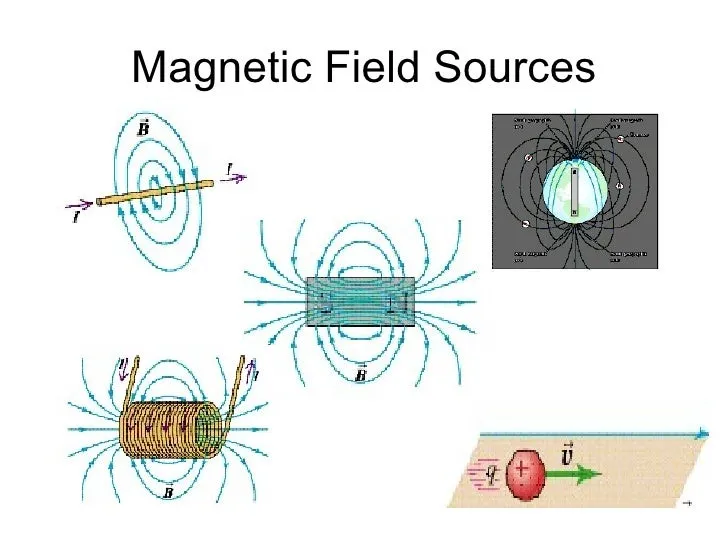## Introduction

Hello it's a me again Drifter Programming! Today we continue with Electromagnetism to get into Exercises all around Magnetic field sources, which we covered during the previous six articles. To cover everything better, we will do 1-2 examples for each topic/article by also getting into the theory again. This means that the post will be a recap about everything that we covered in Magnetic Field sources. So, without further do, let's get started!

## Moving Charged Particle Field

The magnetic field of a single point charge q that is moving with a constant velocity v is given by:(vector and magnitude form)

The component μ0 is the so called permeability of free space, which is connected with the permittivity of free space (ε0) by the equation: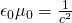with c being the speed of light.

The direction of the produced "circular " magnetic field lines can be found using a right hand rule, which is used for positive charges. For negative charges the force is opposite or we simply use the left hand! :p

By knowing all except one of the following: q, r, v, φ and B, we can find the other very simply by solving the equation...No example needed :D

## Parallel Charged Particles

When putting two of those charges in parallel, each one gets affected by the other one by an electric and magnetic force. The forces applied are:The total force applied is the vector sum of those forces

Another useful equation is the ratio of the forces, which gives us: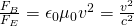and so let's us calculate one of the two forces, when knowing the other...

Let's get into an actual arithmetic Example!

### Example

Let's consider two charges that are moving perpendicular to each other (φ = 90) , with a distance of d = 0.4m on each axis (x and y). Both charges have the same magnitude of charge q = 0.3μC, but of opposite sign. The velocities of those moving charges have the same magnitude v = v' = 6x10^5 m/s^2.

Calculate:

• The electiric force applied on each charge
• The magnetic force applied on each charge
• The total force applied on each charge

1.

Having opposite charge the forces are attractive. The direction of those forces is in the diagonal that has a length of: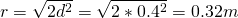That way the forces have the same magnitude which is: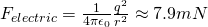2.

Knowing the velocity v, from the "ratio" equation the magnetic force is: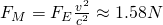3.

The total force is the sum of the previous two and so approximately equal to the magnetic field, which has a "higher" magnitude...## Current-Carrying Conductor Field

The magnetic field produced by current-carrying conductors/wires is forming circles around it that we can find using a similar rule to the previous one, where velocity is being replaced by current. Using the knowledge from charged particles we can proof Biot-Savart's law which is: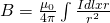For an infinite length wire we can proof that:### Example

Let's consider such a infinite length wire that has a current of I = 100A. We want to have a magnetic field density/magnitude B = 0.5x10^-4T. At which distance does the magnetic field have such a magnitude?
By using the previous equation that we get through Biot-Savart's law we have: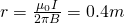## Force Between Parallel Conductors

The force between two parallel currents I1 and I2, separated by distance r is has a magnitude per unit of length given by the equation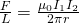This force is attracitve on currents that flow in the same direction and repulsive for currents that flow in opposite directions!

### Example

Let's consider to wires with opposing currents that have the same magnitude of I = 15A. They are of course parallel and straight-line and the distance between them is r = 4.5mm. Calculate the Force/meter ratio.

From the previous equation we have: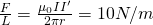By calculating this ratio we can find out if the wire durability has an important role or not in very specific applications.

## Current Loop Field and Force

The magnetic field in the center and axis of a "perfect" current loop can be found by the equations:These two equations can be used in the same way as before and so knowing all except one can help us find the remaining quantity...

## Ampere's Law

The law is defined as: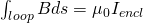or even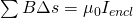and so we calculate a closed loop integral (or sum) of the magnetic field B inside of which a total current Iencl is enclosed. To use this equation you can use any closed path that you want and it's very important that this path is closed!

Actual arithmetic applications are similar to the previous ones and so I just suggest you to go and read the article about that, which had the most important applications...

## Displacement Current

The density of displacemenet current can be found by: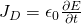where:

• J is the current density
• ε0 the permittivity of free space
• E the electric field (the change by time)

The actual current is defined as:where:

• I is the displacement current
• ε the permittivity (ε = ε0*εr |  electric constant * relative permittivity of dielectric)
• Φ the electric flux (the change of it by time)
For a capacitor the "conductive" current and displacement current have the same magnitude (which can be proven very easily)

We mostly don't use these things directly but together with:

## Ampere's Law Extension

By putting displacement current into the game we end up with the "extended" version of Ampere's law (Maxwell equation) which is: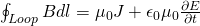By including displacement current we can now analyze magnetic fields produced by charges and also non-charges, that expains the bevahior of capacitors. In the previous post we got into a small application that proved that the current is the same, either we talk about "conductive" or displacement current.

When calculating the magnetic field we do the same as with the "first and simple" Ampere law, but have more component in the right side. The current density J can be found very easily by using the knowledge that we already have from previous articles. The magnetic field or flux per time is also easy to calculate and something that we will get into later on much more in-depth after talking about induction and also alternating current. Getting into an example before knowing the last two doesn't make so much sense, cause the change with time becomes difficult to specify and therefore calculate. But, either way nothing really changes that much when comparing these "new" applications with the "previous" ones...

### REFERENCES:

Mathematical equations that I had in this post where drawn using quicklatex

### Electric fields:

Getting into Electromagnetism -> electromagnetim, electric charge, conductors, insulators, quantization

Coulomb's law with examples -> Coulomb's law, superposition principle, Coulomb constant, how to solve problems, examples

Electric fields and field lines -> Electric fields, Solving problems around Electric fields and field lines

Electric dipoles -> Electric dipole, torque, potential and field

Electric charge and field Exercises -> examples in electric charges and fields

### Electric flux:

Electric flux and Gauss's law -> Electric flux, Gauss's law

Applications of Gauss's law (part 1) -> applying Gauss's law, Gauss applications

Applications of Gauss's law (part 2) -> more Gauss applications

Electric flux exercises -> examples in electric flux and Gauss's law

### Electric potential:

Electric potential energy -> explanation of work-energy, electric potential energy

Calculating electric potentials -> more stuff about potential energy, potential, calculating potentials

Millikan's Oil Drop Experiment -> Millikan's experiment, electronvolt

Cathode ray tubes explained using electric potential -> cathode ray tube explanation

Electric potential exercises (part 1) -> applications of potential

Electric potential exercises (part 2) -> applications of potential gradient, advanced examples

### Capacitance:

Capacitors (Condensers) and Capacitance -> Capacitors, capacitance, calculating capacitance

How to solve problems around Capacitors -> combination, solving problems, simple example

Electric field energy and density -> Electric field energy, energy density

Dielectric materials -> Dielectrics, dielectric constant, permittivity and strength, how to solve problems

Electric capacitance exercises -> examples in capacitance, energy density and dielectrics

### Current, resistance and EMF:

Electric current -> Electric current, current density

Electrical resistivity and conductivity -> Electrical resistivity, conductivity, thermal coefficient of resistivity, hyperconductivity

Electric resistance -> Resistance, temperature, resistors

Electromotive Force (EMF) and Internal resistance -> Electromotive force, internal resistance

Power and Wattage of Electronic Circuits -> Power in general, power/wattage of electronic circuits

Electric current, resistance and emf exercises -> exampes in all those topics

### Direct current (DC) circuits:

Resistor Combinations -> Resistor combinations, how to solve problems

Kirchhoff's laws with applications -> Kirchhoff's laws, how to solve problems, applications

Electrical measuring instruments -> what are they?, types list, getting into some of them, an application

Electronic circuits with resistors and capacitors (R-C) -> R-C Circuit, charging, time constant, discharging, how to apply

RC circuit exercises -> examples in Kirchhoff, charging, discharging capacitor with/without internal resistance

### Magnetic field and forces:

Magnetic fields -> Magnetism, Magnetic field

Magnetic field lines and Gauss's law of Magnetism -> magnetic field lines, mono- and dipoles, Flux, Gauss's law of magnetism

The motion of charged particles inside of a magnetic field -> straight-line, spiral and helical particle motion

Applications of charged particle motion -> CERN, Cyclotrons, Synchrotrons, Cavity Magetron, Mass Spectrometry and Magnetic lens

Magnetic force applied on Current-Carrying Conductors -> magnetic force on current-carrying conductors/wires, proofs

Magnetic force and torque applied on current loops (circuits) -> magnetic force on current loops, magnetic moment and torque

Explaining the Physics behind Electromotors -> tesla, history and explaining the physics behind them

Magnetic field exercises -> examples in magnetic force, magnetic flux, particle motion and forces/torque on current-carrying conductors

### Magnetic field sources:

Magnetic field of a moving charged particle -> moving charge, magnetic field, force between parallel charged particles

Magnetic field of current-carrying conductors -> magnetic field of current, Biot-Savart law

Force between parallel conductors and the magnetic field of a current loop -> force between parallel conductors, magnetic field of current loop

Ampere's law and Applications -> Ampere's law, applications

Magnetic materials -> Magnetic materials, classification and types, material examples

Displacement current -> Displacement current, Extension of Ampere's law

And this is actually it for today's post! Next time in Physics we will start getting into Electromagnetic Induction, together with Faraday's law...

Bye!

H2
H3
H4
3 columns
2 columns
1 column
1 Comment